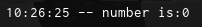# Math.random cant return a number less than 1

i need a math.random that returns a number from 0.02 to 0.08, but it always returns 0. how could I solve it?``````local randomnumber = math.random(0.02, 0.08)
print("number is:" ..randomnumber)
``````

math.random returns integers.

Try using `Random.new():NextNumber()`

Or divide it by 100[ the result]:

``````local randomnumber = math.random(2, 8) / 100
print("number is:" ..randomnumber)
``````

See more here:

2 Likes

Have you tried

``````local randomnumber = math.random(2,8)/100
print("number is:" ..randomnumber)
``````
1 Like

I mean you just kinda write your solution then edit it to include mine

1 Like

No?

But erased it and gave her a nicer method, which is `Random.new():NextNumber()`

People can have the same thought, does that mean they steal it from each other? no.

it worked perfectly, it returned the right number, but I wanted to put the script in a recoil system on my weapon, is it possible to use math.random to use it in CFrame.Angles?

Yeah it may be true that you thought of the idea, but it is a little convenient that 30 secs after my simpler one, you update yours with mine.

Yeah, if you wanted random angles on all 3 axes, just plug it in:

``````local function getSmallRandom()
return math.random(2,8)/100
end

CFrame.Angles(getSmallRandom(), getSmallRandom(), getSmallRandom())
``````

I think you mean like this?

exactly what i neeeeeeeeeeded, tysm!

Just adding into the thread that if you don’t want to rotate it alot, Ensure to use `math.rad` to get the value in radians. Putting the “raw” value itself might not go that well if it is really big/small.

1 Like

Oh yeah sorry I forgot to mention, CFrame.Angles takes in radians, as @MeCrunchAstroX mentioned, use math.rad to convert it to radians.
https://developer.roblox.com/en-us/api-reference/lua-docs/math

Or maybe you wanted it in radiansit’s unlikely though

1 Like